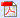## Aug 15, 2009

### Fraction Word Problems

Fraction word problems for addition and subtraction is given here. This fraction word problems worksheet contains both proper and improper fractions. Student needs to be be really careful in understanding the real world situation of fraction word problems, needs to be cautious where to apply addition and subtraction.

1)Stewart won 50 1/5 dollars and Edward won 30 2/5 dollars in a race. What was the total cash price of the race?

Solution:

To find total amount, add 50 1/5 and 30 2/5.
Here the denominators are equal.

50 1/5 + 30 2/5 = 80 3/5.

Total cash prize = 80 3/5 dollars.

2) Mr. Mark’s family went for a trip. To make the journey interesting, they travelled first 53 1/4 km by car and the remaining 10 2/3 km by horse ride. What was the total distance of the trip?

Solution:

To find the total distance, add 53 1/4 and 10 2/3.

Let us convert the given mixed fractions into improper fractions.

53 1/4= 213/4 and 10 2/3 = 32/3.

Here the denominators are unequal.

To make the denominators equal, we have to find the L.C.M of 3 and 4.

Multiples of 3= 3, 6, 9, 12 ….

Multiples of 4= 4, 8, 12, 16 ….

L.C.M= 12

To find common denominator, multiply both numerator and denominator of 213/4 by 3 and 32/3 by 4.

213/4 = 639/12 ; 32/3 = 128/12

Now, 639/12+ 128/12 = 767/12 km = 63 11/12 km

Total distance and covered = 63 11/12 km.

3) Mr. White and Co. has total profit of 6/7 of million dollars. The spinning department gave a profit of 1/2 of million dollars and weaving department gave a profit of 1/4 of million dollars. Find the fraction of profit from other departments.

Solution:

Total profit =6/7
Profit from spinning and weaving departments=1/2 +1/4

Profit from other departments =6/7-(1/2+1/4)

Here to make the denominators equal we have to find the L.C.M of 7, 2 and 4.

Multiples of 7= 7, 14, 21, 28 ….

Multiples of 2= 2, 4, 6, 8, 12, …, 26, 28 …

Multiples of 4= 4, 8, 12, 16, 20, 24, 28 …

L.C.M= 28.

6/7= 6*4/7*4 = 24/28 (To make the denominator 28, multiply and divide by 4).

1/2= 1*14/2*14 =14/28 (To make the denominator 28, multiply and divide by 14).

1/4=1*7/4*7 =7/28 (To make the denominator 28, multiply and divide by 7).

Profit from other departments = 24/28-(14/28+7/28)

= 24/28 - 21/28

= 3/28

Therefore profit from other departments= 3/28 of million dollars.

-----------------------------------------------------------------------------------

Fraction Word Problems Worksheet:

i) Three friends Christ, Marx and Davey participated in gun shooting events. Each of them was given 10 chances. They hit the targets 3 times, 2 times and 4 times respectively out of 10 chances each. Find the total targets hit by them in fraction.
ii) An side of an equilateral triangle is 3/11 feet. Find the perimeter of a triangle.
iii) The length and width of a rectangle is 3/2 cm and 1/2 cm respectively. Find the perimeter of a rectangle.

iv) Sam celebrates his birthday with two small cakes. 7 people attended the birthday party. What fraction of cake each one will receive excluding Sam?

v) Nick and Robert have worked together and completed 3/4 of work in a day. Robert has done 2/5 of work. Find the work done by Nick.

vi) Mr. Briar, principal of Edward Public School, collects funds for School development. 2/3 of funds are collected from school students and 1/6 of funds are collected from school development charity and the rest are collected from Public. What fraction of funds collected from public?

vii) Anne has 1 pizza, Eris ton has 1 3/4 of pizza and Julie has 1/7 of pizza. Find the total number of pizzas.

viii) To make a milk shake, 2 gallons of milk, 1/7 of gallons of essence and 3/4 of gallons of fruit juice were required. Find the total gallons of milk shake made out of it.

ix) Harris has to travel 2 2/3 of a mile. He walked 1 1/3 of the distance and the remaining by car. Find the distance covered by car.
x) Kate and Tony took part in running race. They covered a distance of 5/8 yards and 8/9 yards respectively. Find the distance between these athletes.
Questions:Answers: